# Multiplication Problems

#### Number of problems found: 831

• Francesca 2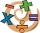Francesca applied the steps below to find the product of (-1.2)(-9.4). Step 1: (-1.2)(-9.4) = (-9.4)(-1.2) Step 2:                   = (-9.4)(-1) + (-9.4)(-0.2) Step 3:                   = (9.4) + (1.88) Step 4:                   = 11.28 Which step shows
• Mother 8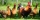Mother bought 2 kg of beef at P240 per kilo and 2 kg of chicken legs at P140 per kilo. She gave the meat vendor a P1000 bill. How much change did she get?
• Mother 7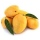Mother bought 18 fruits. 1/3 were pineapple and the rest  were mangoes . how many were mangoesAdd six to twelve, then divide the sum by four. Divide the quotient by negative nine and then multiply by twenty-four. What is the result?
• Gas priceIf a cm3 of gas costs rm 1.50, how many cents would a liter of gas cost? 1 rm = 1 Malaysian Ringgit = 100 Malaysian Ringgit cents = equals 0.21 Euro in 2021/Q3
• If Ben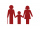If Ben is ⅕ the age of his father, ¼ the age of his mother, and twice the age of his sister, how old are his parents and sister if he is 8 years old?
• Product and sumWhat is the product of two fourths  and the sum of three halves and four?About 6/9 of the sixth- grade pupils will be going to the parents' seminar. If 1/6 of the participants are girls, what part of the portion of sixth graders are boys?
• The libraryThere are 65 boxes of books. Each box contains 100 books. If each book costs 19.75 how much is the total cost of all the books?
• Mistake in expression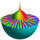While attempting to multiply the expression (2 - 5i)(5 + 2i) a student made a mistake. (2 - 5i)(5 + 2i) = 10 + 4i - 25i - 10i2 = 10 + 4(-1) - 25(-1) - 10(1) = 10 - 4 + 25 - 10 = 21 Complete the explanation and correct the error. Hint: The student inc
• In dividingIn dividing fractions, get the reciprocal of the divisor and change division symbol to multiplication symbol. 2/3 : 5/6
• Nice two numbersWhat two numbers we give a sum of 30 difference of 24 products of 81 and a quotient of 9?
• Central Park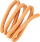Central Park is a rectangular park in New York City and the map scale is 1 cm: 320 m (It means 1 cm on the map represents 320 m in the real world. ). Find the north-south distance if the length on the map is 2.5 cm.
• The space museum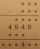The science club is going to the space museum. The club consists of 27 students. If admission costs \$6.50 for children ages 4–11 and \$8.50 for children ages 12–17, how much will it cost for the entire science club's admission to the museum? Science Club S
• Nathan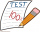Nathan is playing a trivia game. Each correct answer is worth 2 ½ points, and each incorrect answer is worth -¼ points. If he gets 35 questions correct and 32 questions incorrect, how many points does he have? A. 87.5 B. 79.5 C. 75 D. 8
• A giraffe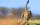A giraffe can run up to 46.93 feet per second. How far could a giraffe run in 1.8 seconds?
• 30 eggs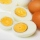There are 30 eggs in a tray. If 1/2 of the tray used 1/5 of it cooked,1/3 kept the refrigerator, how many eggs were left?
• Translate 2Translate the given phrases to mathematical phrases. Thrice the sum of three fifths and two thirds less one half is what number?
• Yellow tangs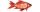In an aquarium 2/5 of the fish are surgeonfish.  Of these 3/4 are yellow tangs.  What fraction of all fish in the aquarium are yellow tangs?
• Three animals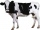Ramrao bought a cow, a buffalo and an ox for a total of rs 85,000. if the ratio of price of cow, buffalo and ox is 4: 8: 5, then what is the price of ox?

Do you have an exciting math question or word problem that you can't solve? Ask a question or post a math problem, and we can try to solve it.

We will send a solution to your e-mail address. Solved examples are also published here. Please enter the e-mail correctly and check whether you don't have a full mailbox.